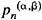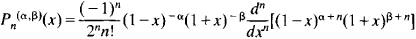# Jacobi Polynomials

Also found in: Wikipedia.

## Jacobi polynomials

[jə′kō·bē ‚päl·ə′nō·mē·əlz]
(mathematics)
Polynomials that are constructed from the hypergeometric function and satisfy the differential equation (1 - x 2) y ″ + [β - α - (α + β + 2) x ] y ′ + n (α + β + n + 1) y = 0, where n is an integer and α and β are constants greater than -1; in certain cases these generate the Legendre and Chebyshev polynomials.

## Jacobi Polynomials

a special system of polynomials of successively increasing degree. For n = 0, 1, 2, . . . the Jacobi polynomials(x) may be defined by the formulaJacobi polynomials are orthogonal on the interval [–1, 1] with respect to the weight function (1 – x)α(l + x)β (seeORTHOGONAL POLYNOMIAL). They were introduced by K. Jacobi in a work published in 1859. Legendre polynomials (α = β = 0), Chebyshev polynomials of the first kind (α = β = –½) and of the second kind (α = β = ½), and ultraspherical polynomials (α = β) are special cases of Jacobi polynomials. Jacobi polynomials are a particular case of the hypergeometric function. They satisfy the differential equation

(1 + x2)y +[β – α – (α + β + 2)x]y +n(α + β +n + 1)y = 0

References in periodicals archive ?
Now, we define the generalized Jacobi polynomials of degree n with a, ff G Z on interval [c, d] by
 , On the zeros of subrange Jacobi polynomials, Numer.
Association of Rodrigues Representation with Jacobi Polynomials
In the theory of classical orthogonal polynomials, the asymptotic relations between Jacobi polynomials and Hermite and Laguerre polynomials are well known.
More recently, the authors  developed some new results related to the Jacobi polynomials for operational matrices.
J-GL-C methods are based on Jacobi polynomials. The Jacobi polynomials are the eigenfunctions of the singular Sturm-Liouville problem ,
As we know, classical Jacobi polynomials  are defined on the interval of [phi] [member of] [-1, 1] and the recurrence formula of the classical Jacobi polynomials [P.sup.([alpha],[beta]).sub.i]([phi]) of degree i is given by
Author Brian George Spencer Doman examines classical orthogonal polynomials and their additional properties, covering hermite polynomials, associated Laguerre polynomials, Legendre polynomials, Chebyshev polynomials, Gegenbauer polynomials, associated Legendre functions, Jacobi polynomials, and many other related mathematical subjects over twelve chapters and appendices.
 proposed a computational method based on Jacobi Polynomials for solving fuzzy linear FDE on interval [0,1].
Pavlovic, "New class of filter functions generated directly by the modified Christoffel-Darboux formula for classical orthonormal Jacobi polynomials", Int.
In this paper by use of shifted Jacobi polynomials as basis and operational matrix of derivatives , of them we convert these kinds of equations to nonlinear algebraic equations.

Site: Follow: Share:
Open / Close